• 偏自相关图跟相关图类似， 横坐标表示延迟阶数，纵坐标表示偏自相关系数 相关图与偏自相关图的python代码实现： from statsmodels.graphics.tsaplots import plot_acf, plot_pacf plot_acf(b.salesVolume) plot...
• 自相关性分析

2015-05-25 10:17:22
matlab中对数据进行相关和偏自相关的数据分析，并作图。
• 详解相关性检验

万次阅读 2020-02-09 21:16:10
相关性检验 使用皮尔逊相关系数和斯皮尔曼相关系数来衡量两个变量间相关性的大小 文章目录(1)描述性统计1.使用Matlab进行计算2.使用Excel计算3.使用Spss计算(2)正态分布的检验0.偏度与峰度1. JB 检验(大样本n>...

相关性检验
使用皮尔逊相关系数和斯皮尔曼相关系数来衡量两个变量间相关性的大小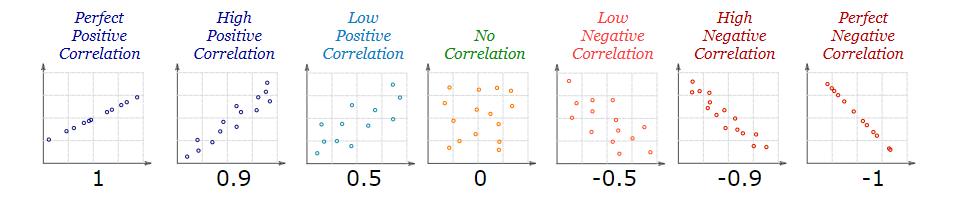(1)描述性统计

• 所谓描述性统计，就是对已有的数据的多个特征(最小元素，最大元素，均值，中位数等)进行计算。

1.使用Matlab进行计算

• min求最小值
• max求最大值
• mean求平均值
• median求中位数的值
• skewness求偏度
• kurtosis求峰度
• std求标准差

2.使用Excel计算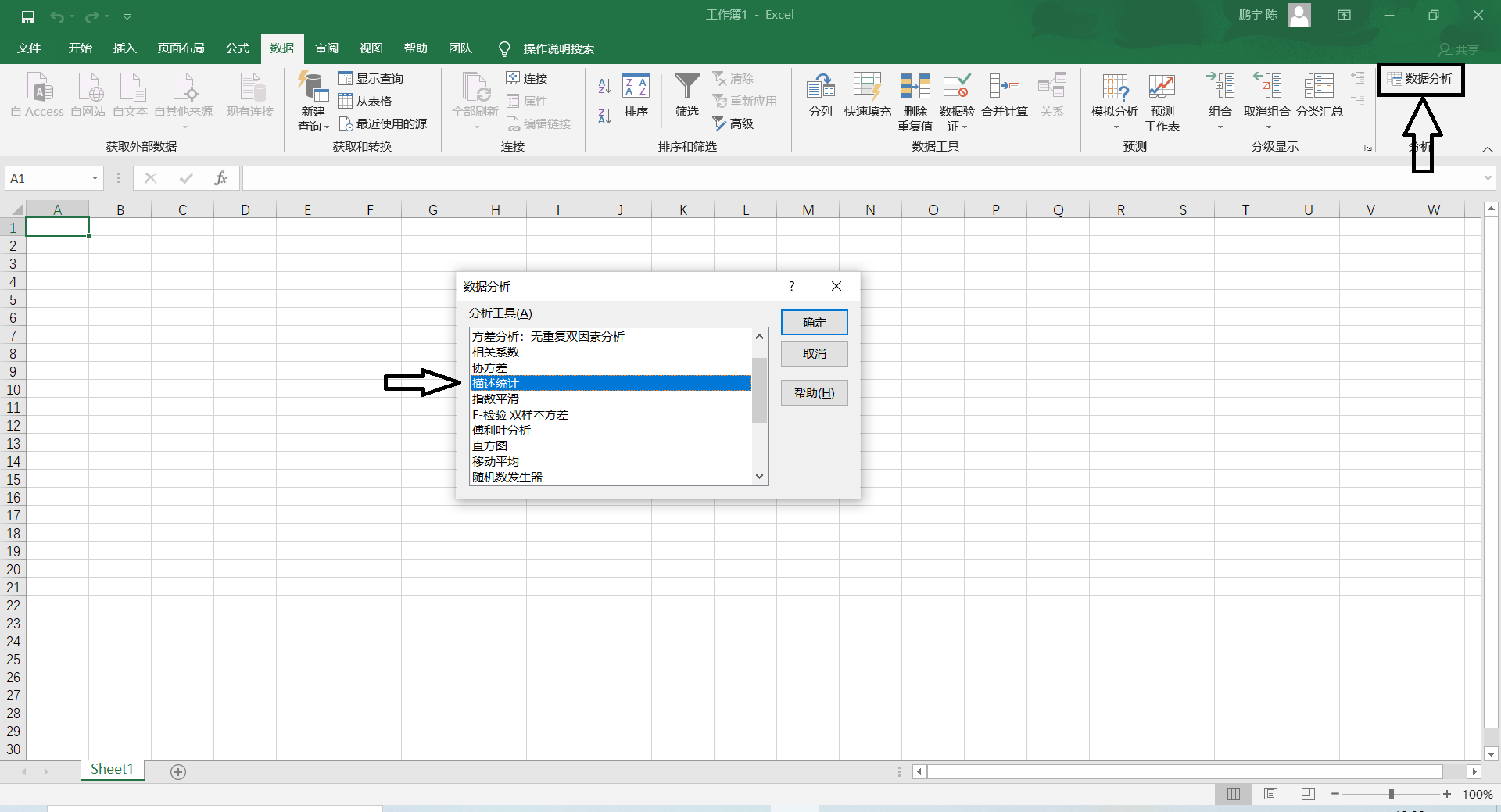3.使用Spss计算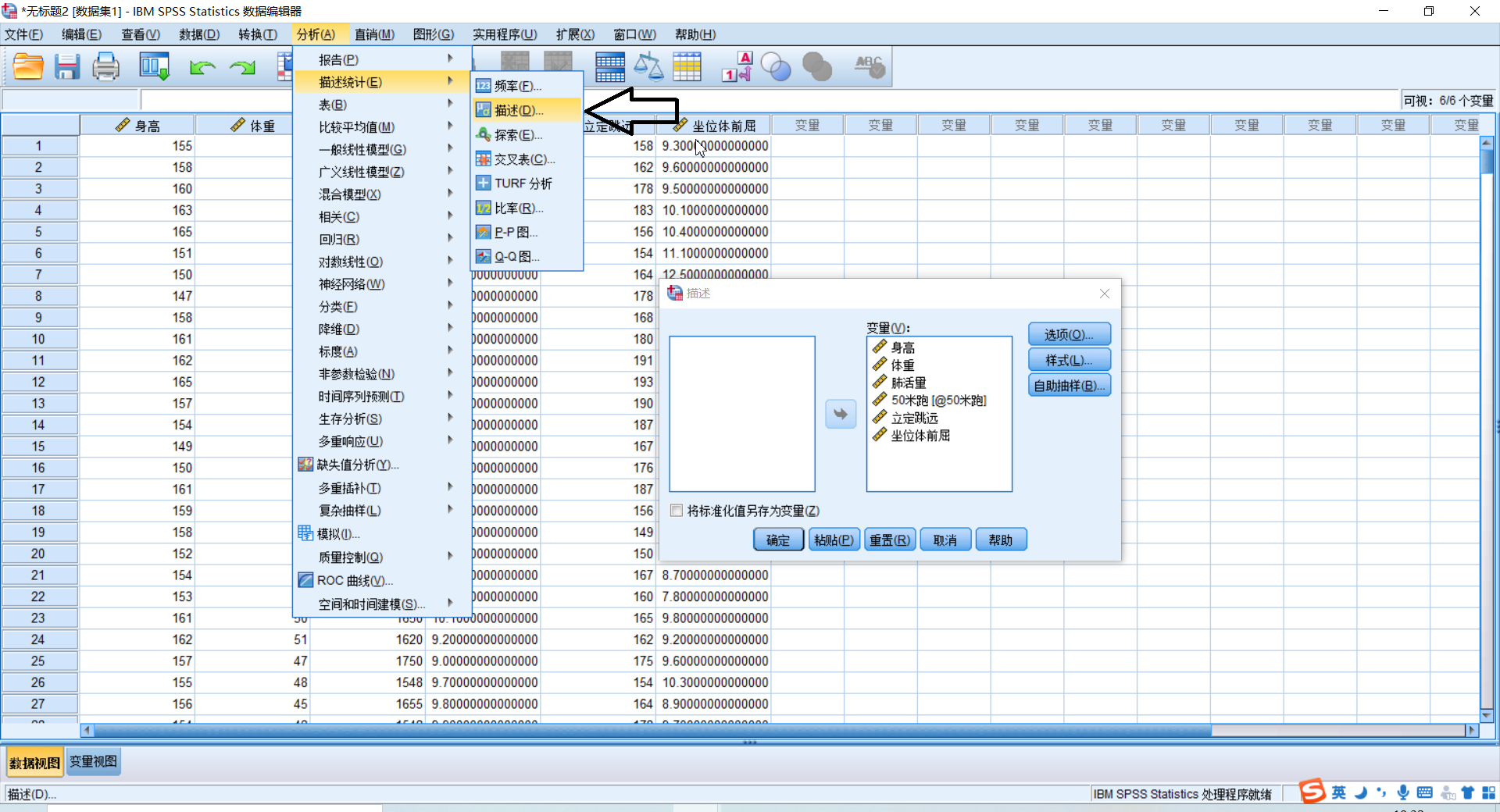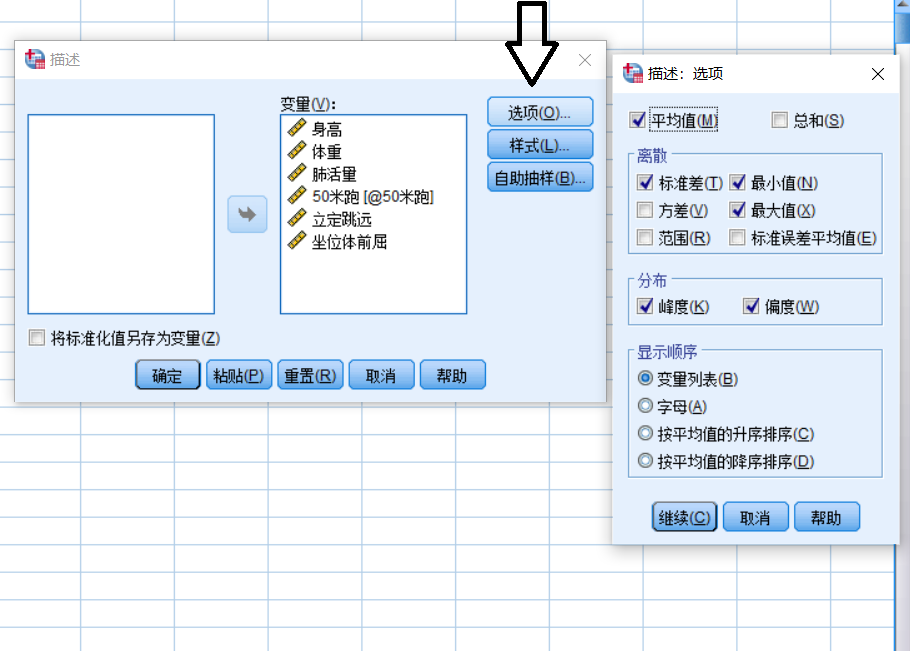(2)正态分布的检验

0.偏度与峰度

• 偏度：是统计数据分布偏斜方向和程度的度量，是统计数据分布非对称程度的数字特征。定义上偏度是样本的三阶标准化矩。
S = E [ ( X − μ σ ) 3 ] S=E[(\dfrac{X-\mu}{\sigma})^3]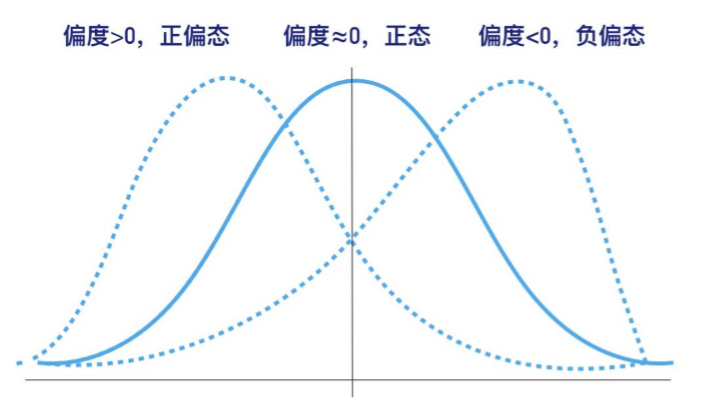注意正态分布的偏度为0

• 峰度：表征概率密度分布曲线在平均值处峰值高低的特征数。直观看来，峰度反映了峰部的尖度。定义上偏度是样本的四阶标准化矩。
K = E [ ( X − μ σ ) 4 ] K=E[(\dfrac{X-\mu}{\sigma})^4]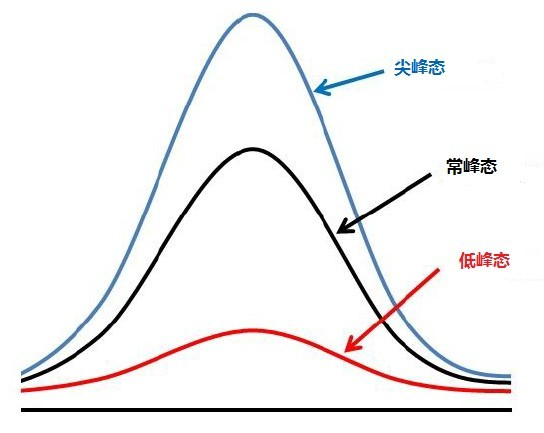注意正态分布的峰度为3

1. JB 检验(大样本n>30)

• step 1：进行假设检验 H 0 : H_0: 该变量服从正态分布， H 1 : H_1: 该变量不服从正态分布。
• step 2：假设对一个随机变量 X i X_i ，其峰度为 K K ，其偏度为 S S ，则构造检验统计量 J B = n 6 [ S 2 + ( K − 3 ) 2 4 ] ∼ χ 2 ( 2 ) JB=\dfrac{n}{6}[S^2+\dfrac{(K-3)^2}{4}]\sim \chi^2(2) 服从自由度为2的卡方分布。
• step 3： 代入数据计算出 J B ∗ JB^* ，通过 J B ∗ JB^* 计算出对应的 p p 值。
• step 4：通过 p p 值检验得出结论。(一般取 p p 0.05 0.05 )。
• matlab中：[h,p]=jbtest(x,alpha), x x 表示要检测的变量，只能取向量，alpha表示显著性水平，h=1表示拒绝原假设，h=0表示接受原假设，p表示返回的p值。

2. 夏皮洛-威尔克检验

• step 1：进行假设检验 H 0 : H_0: 该变量服从正态分布， H 1 : H_1: 该变量不服从正态分布。
• step 2：使用SPSS计算出相应的 p p 值。
• step 3：通过相应的 p p 值进行判断。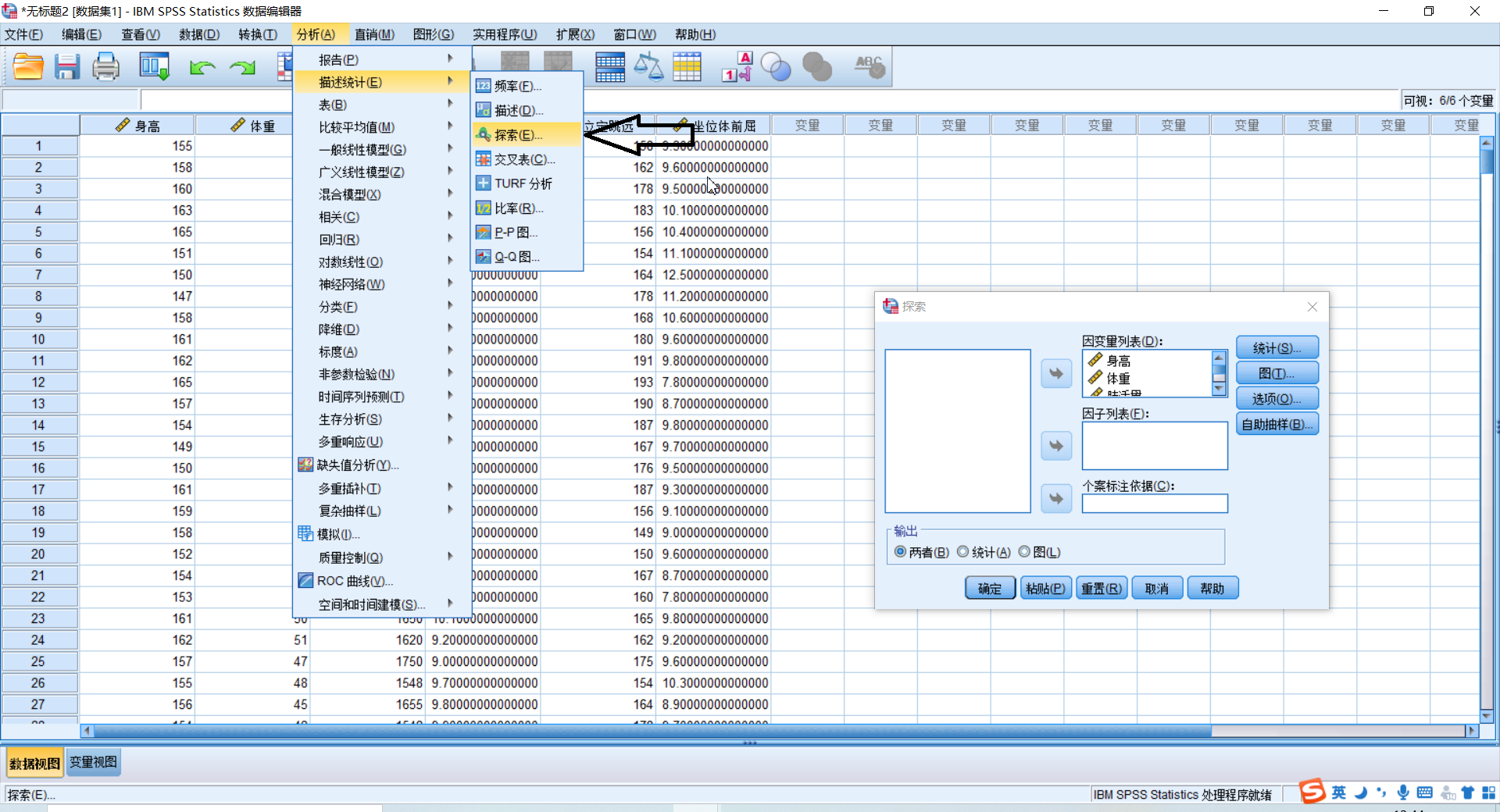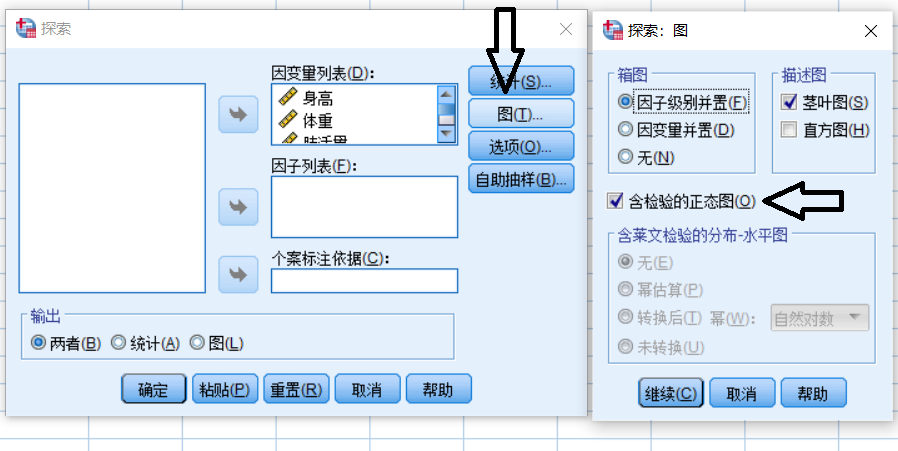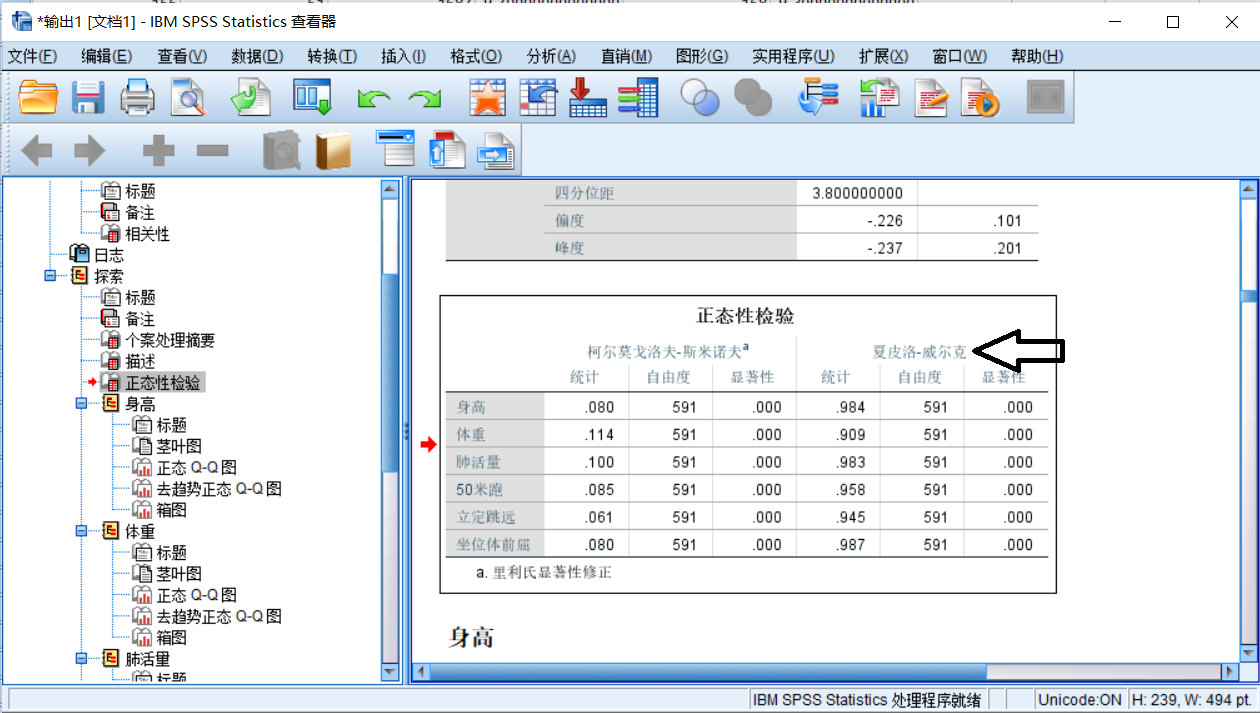(3)皮尔逊相关系数

1.总体皮尔逊Person相关系数

ρ X Y = C o v ( X , Y ) σ x σ y \rho_{XY}=\dfrac{Cov(X,Y)}{\sigma_x\sigma_y}
这里 σ x = ∑ i = 1 n ( X i − E ( X ) ) 2 n \sigma_x=\sqrt{\dfrac{\displaystyle\sum_{i=1}^{n}(X_i-E(X))^2}{n}} σ y = ∑ i = 1 n ( Y i − E ( Y ) ) 2 n \sigma_y=\sqrt{\dfrac{\displaystyle\sum_{i=1}^{n}(Y_i-E(Y))^2}{n}} 分别是 X X 总体与 Y Y 总体的方差。

C o v ( X , Y ) = ∑ i = 1 n ( X i − E ( X ) ) ( Y i − E ( Y ) ) n Cov(X,Y)=\dfrac{\displaystyle\sum_{i=1}^{n}(X_i-E(X))(Y_i-E(Y))}{n} 为总体的协方差。

2.样本皮尔逊Person相关系数

r X Y = C o v ( X , Y ) S x S y r_{XY}=\dfrac{Cov(X,Y)}{S_xS_y}
这里 S x = ∑ i = 1 n ( X i − X ‾ ) 2 n − 1 S_x=\sqrt{\dfrac{\displaystyle\sum_{i=1}^{n}(X_i-\overline{X})^2}{n-1}} S y = ∑ i = 1 n ( Y i − Y ‾ ) 2 n − 1 S_y=\sqrt{\dfrac{\displaystyle\sum_{i=1}^{n}(Y_i-\overline{Y})^2}{n-1}} 分别是 X X 样本与 Y Y 样本的方差。

C o v ( X , Y ) = ∑ i = 1 n ( X i − X ‾ ) ( Y i − Y ‾ ) n − 1 Cov(X,Y)=\dfrac{\displaystyle\sum_{i=1}^{n}(X_i-\overline{X})(Y_i-\overline{Y})}{n-1} 为样本的协方差。

3.使用皮尔逊Person相关系数的注意事项

• 先要判断变量是否大致满足线性关系，可以采用画散点图的方式。当两个变量本来就大致满足线性的关系时，皮尔逊相关系数的绝对值越大，两个变量的相关性越强，但是如果本来就不满足线性关系，就算皮尔逊系数很大也没有意义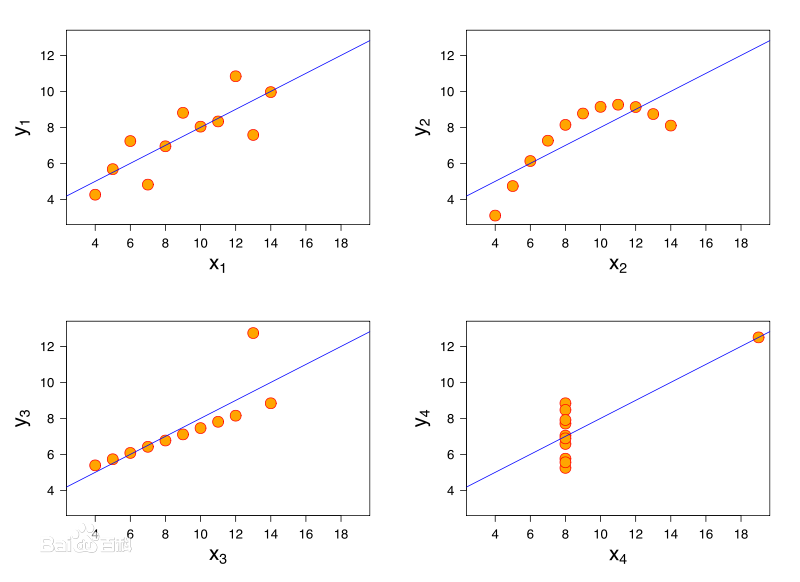上面的几个图的皮尔逊相关系数都为0.816，但是明显小样本对最终结果产生巨大的影响。

• 如果计算出皮尔逊系数为0，不代表两个变量之间不存在关系。比如下面的温度-冰糕之间必然存在相关性，但是不是线性关系。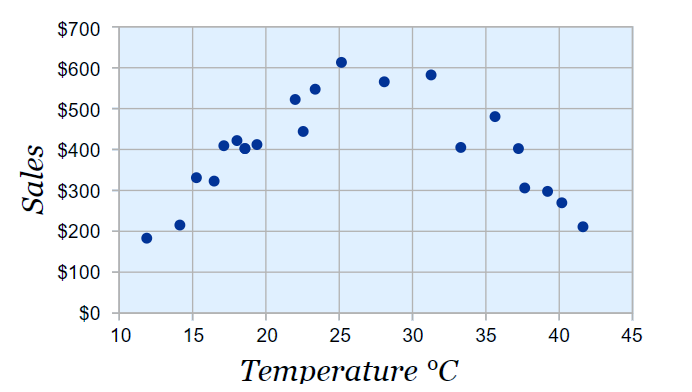• 可以使用 SPSS 作出散点图先判断是否具有一定的线性关系。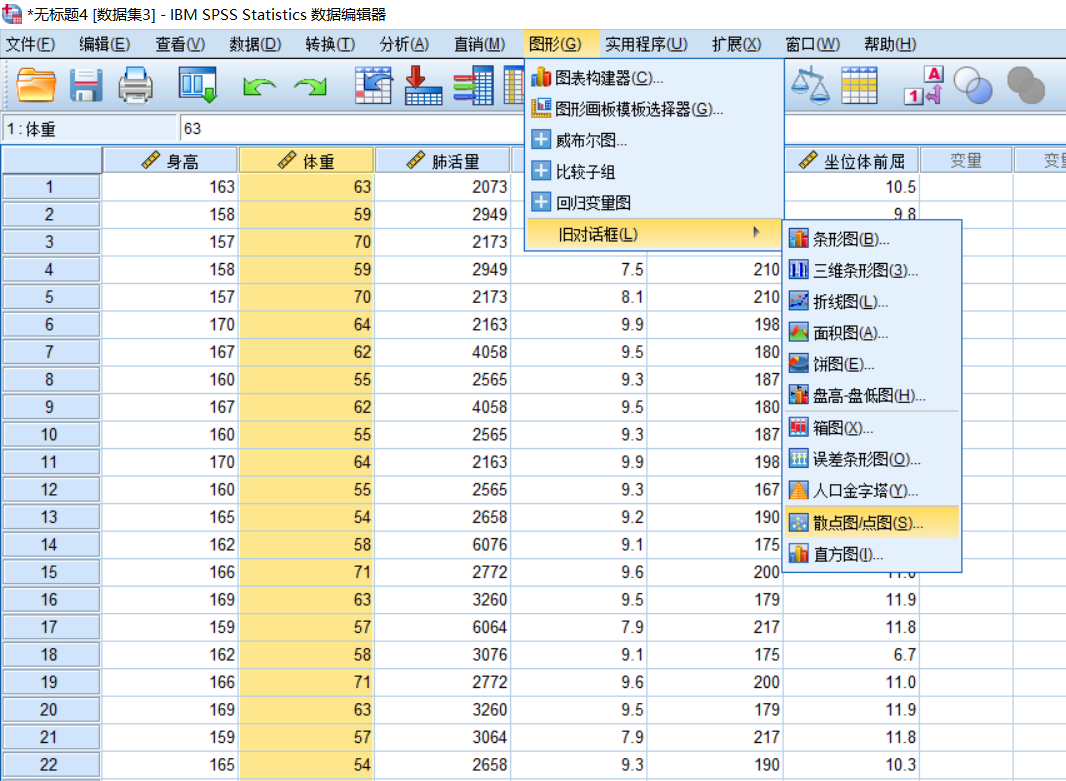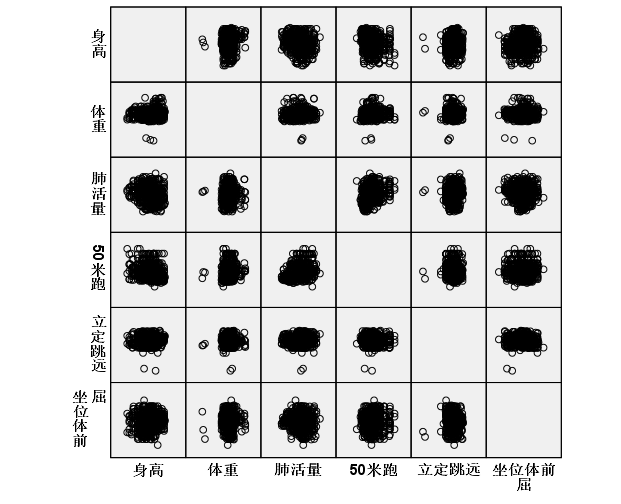4.使用matlab计算person系数

① ① 使用matlab计算
corrcoef

• R=corrcoef(A)：返回 A A 的相关系数矩阵，其中 A A 的列表示随机变量(指标，例如身高、体重等),行表示观测值(样本，例如每一个人的身高、体重等)。
• R=corrcoef(A,B)：返回两个变量 A ， B A，B 向量之间的相关系数。

② ② 使用Excel计算与美化相关系数表

• 在数据分析中选取相关系数的计算。
• 使用色阶图进行美化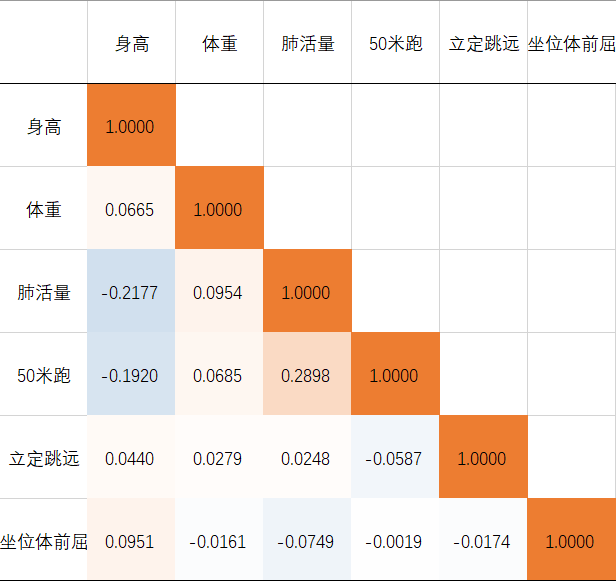(3)对皮尔逊相关系数进行假设检验

1.可以进行假设检验的前提

• 实验数据通常假设是成对的来自于正态分布的总体。(一般情况下很难满足)。
• 实验数据之间的差距不能太大，异常值对检验结果影响很大。
• 每个样本之间是独立抽样的。

3.流程

⭐️目的是验证计算得出的皮尔逊相关系数是否与0有显著的差异。

• step 1 提出原假设与备择假设, H 0 : r = 0 , H 1 : r ≠ 0 H_0:r=0,H_1:r\not=0

• step 2 构造检验统计量
r n − 2 1 − r 2 ∼ t ( n − 2 ) r\sqrt{\dfrac{n-2}{1-r^2}}\sim t(n-2) 服从自由度为2的 t t 分布

• step 3 计算出检验值(代入数据)得到 t ∗ t^*

• step 4 使用 p p 检验

• 计算 p p
p = 2 × ( 1 − ∫ − ∞ t ∗ t ( x ) d x ) p=2\times(1-\int_{-\infty}^{t^*}t(x)dx)
• step 5 结果说明

• p < 0.01 p<0.01 ：在 99 % 99\% 的置信水平上拒绝原假设。
• 0.01 < p < 0.05 0.01<p<0.05 ：在 99 % 99\% 的置信水平上无法拒绝原假设，但在 95 % 95\% 的水平上可以拒绝原假设。
• 0.05 < p < 0.1 0.05<p<0.1 ：在 95 % 95\% 的置信水平上无法拒绝原假设，但在 90 % 90\% 的水平上可以拒绝原假设。
• 显著性标记: a , a ∗ , a ∗ ∗ a,a^*,a^{**} ,** 表示在0.01级别（双尾）的相关性显著，* 表示在0.05级别（双尾）的相关性显著。

• matlab相关:

• tpdf(x,n) x x 取一系列连续的值，可以做出自由度为 n n t t 分布图像。
• tcdf(t,n)：是自由度为 n n t t 分布的分布函数。 t t 取一个固定的值 t c t_c ,可以计算出 T ≤ t c T\le t_c 的概率。
• tinv(p,n)：自由度为 n n t t 分布下对应概率为 p p 的点的值。

(4)斯皮尔曼(sperman)相关系数

1. 斯皮尔曼相关系数的第一种定义

• X X , Y Y 为两组数据，其斯皮尔曼(等级)相关系数：
r s = 1 − 6 ∑ i = 1 n d i 2 n ( n 2 − 1 ) r_s=1-\dfrac{6\displaystyle\sum_{i=1}^{n}d_i^2}{n(n^2-1)} d i d_i X i X_i Y i Y_i 之间的等级差。( − 1 ≤ r s ≤ 1 -1 \le r_s \le 1 )。
• 等级：将所在列的元素排序后，这个数所在的位置。
• 有数值相同，将它们所在位置取算术平均。例如对下面一列数 90 , 84 , 76 , 71 , 71 , 71 , 69 , 68 , 66 , 64 90,84,76,71,71,71,69,68,66,64 排出的顺序为 1 , 2 , 3 , 5 , 5 , 5 , 7 , 8 , 9 , 10 1,2,3,5,5,5,7,8,9,10 计算方式为 4 + 5 + 6 3 \dfrac{4+5+6}{3}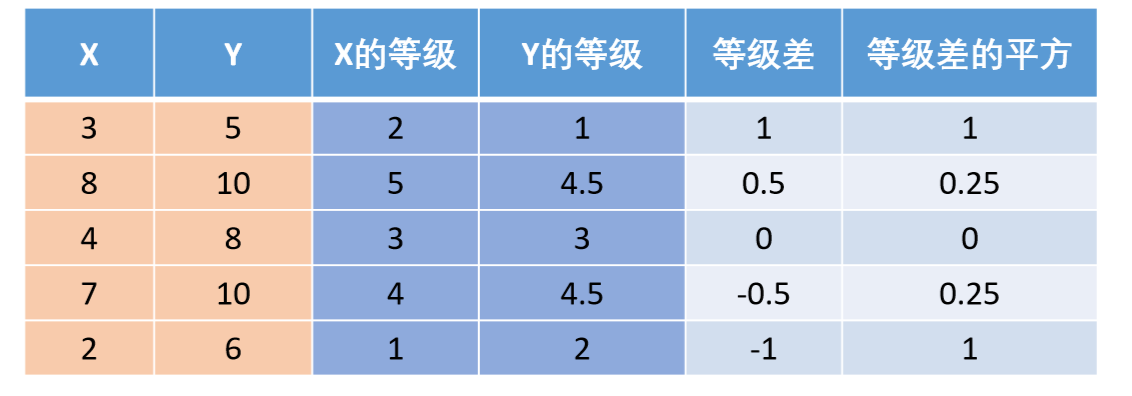2. 斯皮尔曼相关系数的第二种定义

• 定义为等级之间的皮尔逊相关系数
• 按这种定义计算得到的斯皮尔曼相关系数一般与第一种定义下计算出的斯皮尔曼相关系数不同。

3.使用matlab计算斯皮尔曼相关系数

• corr(X,Y,'type','Spearman')：其中 X X Y Y 必须是列向量。
• corr(X,'type','Spearman')：计算矩阵 X X 各列之间的斯皮尔曼相关系数。

(5)斯皮尔曼相关系数的假设检验

H 0 : r s = 0 , H 1 : r s ≠ 0 H_0:r_s=0,H_1:r_s\not=0

1.小样本情况下 ( n ≤ 30 ) (n\le30)

直接在临界表中查询即可。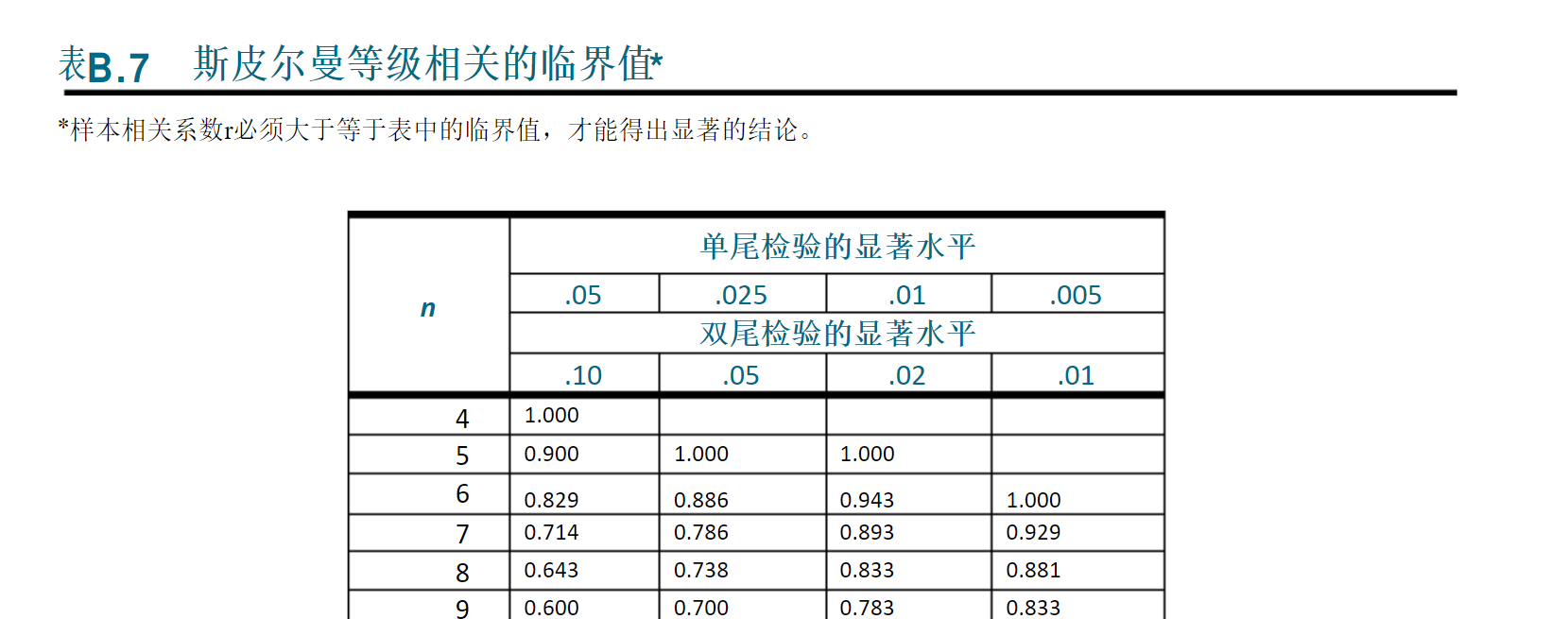2.大样本情况下 ( n ≥ 30 ) (n\ge30)

• 统计量 r s n − 1 ∼ N ( 0 , 1 ) r_s\sqrt{n-1}\sim N(0,1)

• 计算检验值 r s n − 1 r_s\sqrt{n-1}

• 计算对应的 p p 值,再进行判断。

(6)person与spearman对比

• 连续数据，正态分布，线性关系，最好使用 person。
• 除此之外都使用 spearman。
• 两个定序数据之间也只能用spearman。
定序数据：仅仅反映观测对象的等级顺序关系的数据。(例如：优，良，差等)。
展开全文数学建模
• 一、向量回归检验 1. 向量回归检验(VAR)：Vector autoregression 单独建一个AR模型，不足以说明每个变量的影响。此时就需要做VAR模型。 VAR模型针对的是平稳性序列，如果是非平稳性的 ，实质上则进行的是...

一、基本概念

1. 对于模型 y t = b 0 + b 1 x 1 t + b 2 x 2 t + ⋯ + b k x k t + u t y_{t}= b_{0} +b_{1}x_{1t}+b_{2}x_{2t}+\cdots+b_{k}x_{kt}+u_{t} 如果随机误差项 u t u_{t} 的各期望值之间存在着相关关系，即
C o v ( u i , u j ) = E ( u i u j ) 不 等 于 0 ( i 不 等 于 j ) Cov(u_{i},u_{j})=E(u_{i}u_{j}) 不等于0 (i不等于j)
这时，称随机误差项之间存在自相关性（autocorrelation）或序列相关。
2. 随机误差项的自相关性可以有多种形式，详情见https://editor.csdn.net/md/?articleId=114406861。其中最常见的类型是随机误差项之间存在一阶自相关性或一阶自回归形式。
3. 一阶自相关性或一阶自回归，记为AR(1)，即随机误差项只与它的前一期值相关： C o v ( u t , u t − 1 ) = E ( u t u t − 1 ) = / = 0 ( i 不 等 于 j ) Cov(u_{t},u_{t-1})=E(u_{t}u_{t-1}) =/=0 (i不等于j)
或者
u t = f ( u t − 1 ) = α u t − 1 + v t ( − 1 < α < 1 ) u_{t}=f(u_{t-1})=\alpha u_{t-1}+v_{t} (-1<\alpha<1)
则称这种关系为一阶自相关。 α \alpha 称为一阶自相关系数。
4. P阶自相关性可以表示为
u t = a 1 u t − 1 + a 2 u t − 2 + a 3 u t − 3 + ⋯ + a p u t − p + v t u_{t}= a_{1} u_{t-1}+ a_{2} u_{t-2} + a_{3} u_{t-3} + \cdots +a_{p} u_{t-p} + v_{ t}
称之为p 阶自回归形式，记为AR(p），或模型存在 p 阶自相关。

自回归模型
如果滞后的变量模型的解释变量仅包括自变量X的当期值和被解释变量的若干期滞后值，模型的形式为
Y t = α + β X t + a 1 Y t − 1 + a 2 Y t − 2 + ⋯ + a p Y t − p + u t Y_{t} = \alpha +\beta X_{t}+a_{1}Y_{t-1}+ a_{2}Y_{t-2}+\cdots+ a_{p}Y_{t-p}+u_{t}
式中，q为自回归模型的阶数，这类模型称为自回归模型。

1. 由于无法观察到误差项 u t,只能通过残差项 e t来判断 u t 的行为。

2. 通俗讲：自相关无非就是为了了解某个系列（比如股价）受到过去所有/历史上此系列的线性影响，如果模型合适了便可以做预测。

二、向量自回归检验（时间序列模型需要存在自相关）（Eviews）

1. 向量自回归检验(VAR)：Vector autoregression

• 单独建一个AR模型，不足以说明每个变量的影响。此时就需要做VAR模型。
• VAR模型针对的是平稳性序列，如果是非平稳性的 ，实质上则进行的是Johansen协整检验。

2. 步骤：1. 先做平稳性检验。unit root test
如果不平稳，则先转化成平稳的（可采用差分，或者取对数等）。

2. 若平稳，则确定滞后阶数P。
带* 表示最优的阶数。

3. 外生性检验（格兰杰检验）

确定滞后值是否对被解释变量是否有预测能力。若有则进行后续操作，如无则后续操作无意义。若外生检验通过，则表明其他滞后值对当前被解释变量有预测能力，就可以进行模型稳定性的判断。

1. 判断模型的稳定性；（两种发法）若模型稳定，则进行脉冲检验。否则后续检验无意义。

2. 脉冲响应和方差分解（分析各变量的影响）
进入脉冲模型的两种方法：方差分解：三 、 线性回归模型的自相关性检验（回归模型不能存在自相关性）

- 自相关性产生的原因：

1. 经济变量惯性的作用引起随机误差项自相关
2. 经济行为的滞后性引起随机误差项自相关
3. 一些随机因素的干扰或影响引起随机误差项自相关
4. 模型设定误差引起随机误差项自相关
5. 观测数据处理引起随机误差项序列相关

线性回归模型中随机误差项存在序列相关的原因很多，但主要是经济变量自身特点、数据特点、变量选择及模型函数形式选择引起的。

- 自相关的后果

1. 自相关不影响OLS估计量的线性和无偏性，但使之失去有效性
2. 自相关的系数估计量将有相当大的方差
3. 自相关系数的T检验不显著
4. 模型的预测功能失效

线性相关模型的随机误差项存在自相关的情况下，用OLS（普通最小二乘法）进行参数估计，会造成以下几个方面的影响。
从高斯-马尔可夫定理的证明过程中可以看出，只有在同方差和非自相关性的条件下，OLS估计才具有最小方差性。当模型存在自相关性时，OLS估计仍然是无偏估计，但不再具有有效性。这与存在异方差性时的情况一样，说明存在其他的参数估计方法，其估计误差小于OLS估计的误差；也就是说，对于存在自相关性的模型，应该改用其他方法估计模型中的参数。

- 线性自相关性的检验（SPSS）

残差统计和标准化残差图是为了能够直观地看出误差项是否自相关。一阶线性自相关可以使DW-检验。详情见https://editor.csdn.net/md?articleId=113869409

展开全文• 做的是现金股利影响因素的 研究 做了相关分析 但是看不懂图啊啊这个跟普通的相关分析表格解读方法类似。。无非是前面有没有控制变量，有控制变量的，就是当把控制变量控制，或者说的直白点，就是当把这个变量的...

做的是现金股利影响因素的 研究 做了偏相关分析 但是看不懂图啊啊

这个跟普通的相关分析表格解读方法类似。。无非是前面有没有控制变量，有控制变量的，就是当把控制变量控制，或者说的直白点，就是当把这个变量的变化影响剔除后.

下面我要进行偏相关分析了，那么我要怎么进行偏相关分析呢？？？

进行偏相关分析的变量必须是正态分布，各因素之间应该有关联。如果不满足上述条件应该进行转换。在spss的analyze-correlate-partial correlations打开，将两个或两个以.

浓度即为因变量，后面的地点，天气状况，风力，检测时间，温度，适湿度，为变量。那么做偏相关分析，需要控制一个变量，比如说，控制地点，来测定天气状况和风力.

作为控制变量，然后对A和B进行偏相关分析。提问：(1)这样的方式是否可。

偏相关分析文献非常多你这个涉及到重复测量，应该考虑重复测量资料分析方法，或者其他嵌套性数据的处理方法

这个首先要看你的变量数据是否都属于连续性数据，如果都是连续性数据，然后绘制. 如果完全不符合的话 那就只能用其他的来分析，如果只是略微偏态 还是可以用.

当然可以，把要控制的变量选人协方差框，其他步骤同相关分析

比如我对两组的变量分别作偏关分析，然后对这两组做典型相关分析，发现得。

抱歉，刚看到你的求助。结论不一致很正常啊，因为两种分析方法侧重点不同。偏相关分析是控制其他变量，测量两个变量之间的相关性，而典型相关分析是先通过线性组.

matlab偏最小二乘分析(2009-11-20 16:43:07)转载▼标签：杂谈单因变量function y=pls(pz)[row,col]=size(pz);aver=mean(pz);stdcov=std(pz)； %求均值和标准差rr=corrcoef(.

多个变量进行分析，用偏相关分析结果显示是正相关，用双变量分析出来的结。

偏相关和简单双变量相关结果符号相反是正常的，回归分析结果也是如此，因为偏相关和回归分析涉及多个变量，而多个变量的分析暗含着控制其他变量之后再分析特定变.

想分析热效率与其12种影响因素的相关性，因变量应该是热效率，控制变量应.

你好！进行偏相关分析的变量必须是正态分布，各因素之间应该有关联。如果不满足上述条件应该进行转换。在spss的analyze-correlate-partial correlations打开，将两个或.

书中最多只看到控制2个变量，希望得到回复

“偏相关”过程计算偏相关系数，该系数在控制一个或多个附加变量的效应的同时描述 两个变量之间的线性关系。相关是对线性相关性的测量。两个变量可以完全相关，但.

请仔细点说明一下原因和其中的关键概念，万分感谢。另外，逐步回归分析中。

偏相关也叫净相关，其原理是控制(实质是将无关变量与研究关注的变量的相关减去)某一些你不关注但是有可能对你的研究变量有影响的无关变量的影响，来探讨你的研.

偏相关是在有其他变量影响下，将其他变量的影响剔除出去，单纯的求两个变量的关系，可以说这个才是两个变量之间的真正相关性线性与非线性的区别是，线性的话 可以.

y和X1无相关，但是与X2、X3、X4.相关，在偏相关中排除X2、X3、X4后，.

自变量很多的话先进行主成分分析，筛选自变量，再建立回归模型。

偏相关系数的计算可以有下面的三种方法(详细的计算方法见参考文章)1 根据上面的说法，从线性回归的角度计算变量间的偏相关系数，但是这样做很麻烦。2 迭代法，.

朋友，你这个数据可采用pearson相关分析就可以，spss的步骤如下：1、单击analyze——correlate——bivariate.，则弹出相关分析bivariate correlations对话框2、把左边.

研究在多变量的情况下，当控制其他变量影响后，两个变量间的直线相关程度。又称净相关或部分相关。例如，偏相关系数 r13.2表示控制变量x2的影响之后，变量 x1和变.

我们算回归时，要建立数学模型！无论直线或曲线，都要求系数。而这系数就要求合理。最小二乘法就是求偏相关系数并保证其偏差平方和最小。

是的。显著性0.141>0.05，相关性系数不显著，无统计学意义，也可以说是不相关。

展开全文• 01 引言 金融数据主要分为时间序列（时间维度）、横截面（个体维度）和面...金融时间序列分析是量化投资建模的重要基础，今天给大家分享时间序列的一些基础概念，包括自相关性偏自相关性、白噪声和平稳性，以及Py...

01 引言

金融数据主要分为时间序列（时间维度）、横截面（个体维度）和面板数据（时间+截面）。比如上证综指2019年1月至今的日收盘价数据就是时间序列，而2019年8月12日所有A股收盘价数据则是横截面数据，2018-2019年3000多只个股收盘价数据便是面板数据。金融时间序列分析是量化投资建模的重要基础，今天给大家分享时间序列的一些基础概念，包括自相关性、偏自相关性、白噪声和平稳性，以及Python的简单实现，为后续关于时间序列建模专题做一个铺垫。Python中的statsmodels包提供了强大的统计和计量建模函数，其中子模块tsa（time series analysis）专门用于时间序列分析。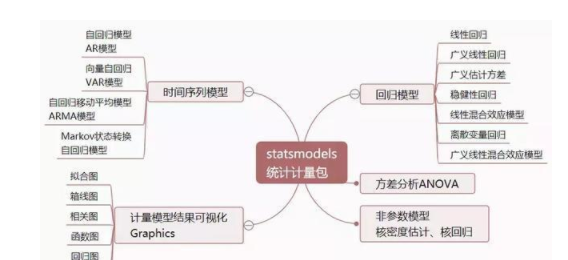02 自相关性

相关性一般是指两个变量之间的统计关联性，那么自相关性则是指一个时间序列的两个不同时间点的变量是否相关联。时间序列具有自相关性是我们能够进行分析的前提，若时间序列的自相关性为0，也就是说各个时点的变量不相互关联，那么未来与现在和过去就没有联系，根据过去信息来推测未来就变得毫无根据。时间序列的自相关性一般用时间序列的自协方差函数、自相关系数函数和偏自相关系数函数等统计量来衡量。遇到问题没人解答，小编创建了一个Python学习交流裙：五二八 三九七 六一七， 寻找有志同道合的小伙伴，互帮互助，群里还有不错的学习视频教程和PDF电子书分享！

自协方差函数

自协方差（Autocovariance,简称AF）是时间序列与其滞后项的协方差，假设X为随机变量（即随着时间变化取值随机的变量,比如股票价格），则k阶自协方差使用数学公式表示为：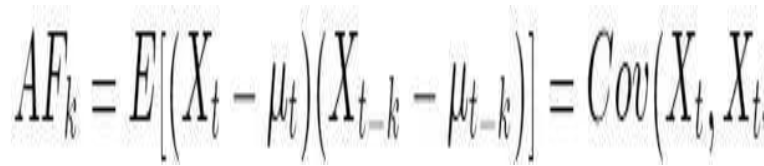其中，E表示求数学期望，u是随机变量X的均值，当k=0时，可得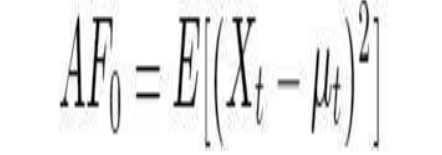即为随机变量X的方差。

自相关函数

自协方差跟变量的单位有很大关系，比如X放大10倍，则自协方差将放大100倍，因此其值大小并不能反映相关性的大小。为了消除量纲（单位）的影响，使用自相关系数来刻画变量与其滞后项的相关性。自相关系数（Autocorrelation Coefficient，简称ACF）本质是相关系数，等于自协方差除以方差，k阶自相关系数数可以表示为：

上过高中数学的都知道协方差和相关系数的含义，从统计上描述两个不同变量的相互影响关系（非因果），那么自协方差和自相关系数则是刻画同一个变量在不同时期取值的相关程度，比如描述上证综指过去价格对今天价格的影响。

偏自相关函数

假设对于上证综指价格序列，一阶自相关系数大于0，说明今天的价格与昨天的价格相关，而昨天价格又与前一日价格相关，依次类推，可见当你计算今天与昨天价格之间的自相关系数时，同时包含了更早之前所有各期的信息对今天的间接影响，度量的是过去所有信息加总的影响效果。为了剔除其他各期的影响，单纯考察过去某一单期对今天的影响，引入偏自相关函数（Partial Autocorrelation Coefficient，简称PACF），即条件自相关系数，使用数学公式表示为：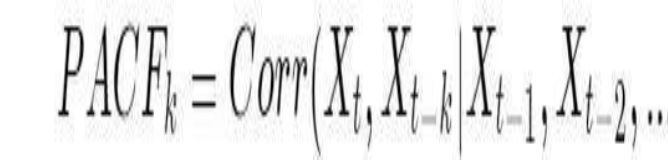偏自相关函数可以通过自回归模型（后续关于时间序列建模会进一步分析）来表述和求解，用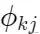表示k阶自回归式中第j个回归系数，则k阶自回归模型表示为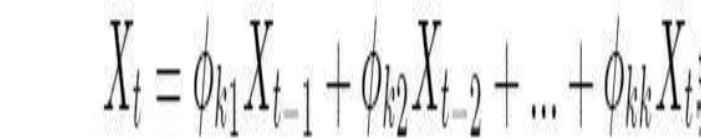其中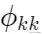是最后一个系数。若把看作滞后期k的函数，则称，k = 1,2… 为偏自相关函数。

自相关系数和偏自相关系数越大，说明过去对现在的影响越大。

Python计算自相关和偏自相关系数

Python的pandas库提供了计算基本统计量的函数，包括均值df.mean(),协方差df.cov(),相关系数df.corr(),方差df.var()（或标准差df.std()）等,其中df为数据列表；而自相关系数和偏自相关系数的计算则要用到statsmodels库（acf()和pacf()）。statsmodels这是一个很强大的统计数理模型库，在后面的时间序列分析与建模会进一步介绍相关函数及其运用。

import pandas as pd

import numpy as np

import matplotlib.pyplot as plt

import matplotlib as mpl

%matplotlib inline

#正常显示画图时出现的中文和负号

from pylab import mpl

mpl.rcParams['font.sans-serif']=['SimHei']

mpl.rcParams['axes.unicode_minus']=False

#获取数据，从tushare上获取沪深300指数为例

import tushare as ts

token='输入你的token'

pro=ts.pro_api(token)

def get_data(code,n=250*3):

df=pro.index_daily(ts_code=code)

#将日期设置为索引

#最近n日价格走势

df=df.sort_index()[-n:]

#只保留收盘价数据

del df.index.name

return df.close

df=get_data('000300.SH')

计算自相关和偏自相关系数

import statsmodels.tsa.api as smt

#tsa是Time Series analysis缩写

#使用help(smt.stattools.acf)可以查看相关参数设置

#计算自相关系数，这里设置滞后项为5期,默认是40期滞后

acf=smt.stattools.acf(df,nlags=5)

#计算偏自相关系数

pacf=smt.stattools.pacf(df,nlags=5)

print(f'自相关系数为：{acf};\n偏自相关系数为：{pacf}')

自相关系数为：[1. 0.99098764 0.98189466 0.97312885 0.96252012 0.95335064];

偏自相关系数为：[ 1. 0.99231072 -0.01047826 0.01620047 -0.12635305 0.09200772]

自相关系数和偏自相关系数可视化

def acf_pacf_plot(data, lags=None):

#判断是否为pandas的Series格式数据

if not isinstance(data, pd.Series):

data = pd.Series(data)

#设定画面风格，这里设置为'bmh', colspan=2

with plt.style.context('bmh'):

fig = plt.figure(figsize=(10, 8))

#设置子图

layout = (3,1)

ts_ax = plt.subplot2grid(layout, (0, 0))

acf_ax = plt.subplot2grid(layout, (1, 0))

pacf_ax = plt.subplot2grid(layout, (2, 0))

data.plot(ax=ts_ax)

ts_ax.set_title('时间序列图')

smt.graphics.plot_acf(data, lags=lags, ax=acf_ax, alpha=0.5)

acf_ax.set_title('自相关系数')

smt.graphics.plot_pacf(data, lags=lags, ax=pacf_ax, alpha=0.5)

pacf_ax.set_title('偏自相关系数')

plt.tight_layout()

return

#设置20阶滞后期

acf_pacf_plot(df,lags=20)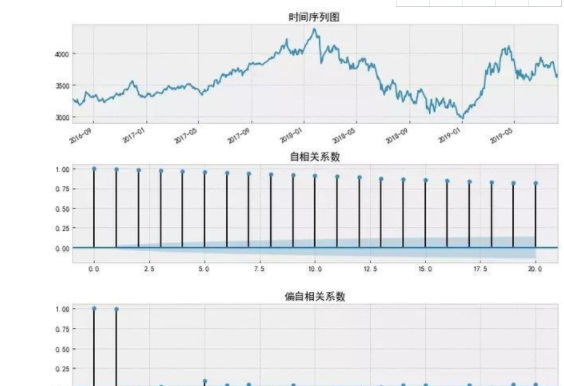03 平稳性

时间序列分析的主要目的是利用事物特征变量的历史和现状来推测未来可能出现的状况，即假设时间序列的基本特性必须能从过去维持到我们推测的时期，否则，基于历史和现状来预测未来将变得不可靠。时间序列的平稳性，简单理解是时间序列的基本特性维持不变，换句话说，所谓平稳性就是要求由样本时间序列所得到的曲线在未来的一段时期内仍能沿着现有的形态持续下去。金融领域很多变量之所以难以估计，是因为这些变量经常发生突变，不是平稳的时间序列。时间序列的平稳性是经典时间序列分析的基本假设前提，只有基于平稳的时间序列进行的预测才是有效的。平稳性有强平稳和若平稳之分，一般所说的平稳时间序列指的是若平稳时间序列。

强平稳（Strictly Stationary）

强平稳要求时间序列随着时间的推移，其统计性质保持不变，对于任意的τ，其联合概率密度函数满足：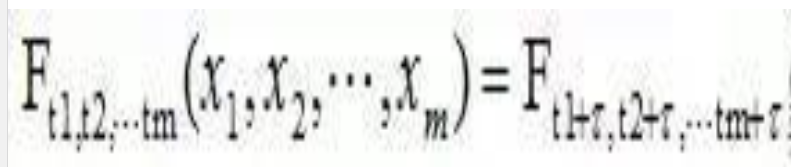，则时间序列是强平稳的。

强平稳是一个很强的条件，要求该时间序列的任何统计性质都不会随着时间发生变化。强平稳由于条件苛刻，理论和实证上都难以检验，因此现实中几乎无法运用。

弱平稳（Weakly Stationary）

弱平稳放宽了平稳性条件，只要求低阶矩平稳，即数学期望（均值）和方差不随时间和位置变化。弱平稳过程的条件是：

（1）均值函数在所有时间上恒为常数；

（2）存在二阶矩；

（3）对于所有时间t和和时滞k，自协方差相同。

值得注意的是，强平稳和弱平稳时间序列二者并没有包含关系。换句话说，强平稳时间序列不一定是弱平稳的，因为强平稳过程不一定存在二阶距；弱平稳时间序列也不一定是强平稳过程，因为弱平稳只能保证一阶矩和二阶距不随时间变化，但不能保证其有穷维分布不随时间变化。弱平稳的正态分布时间序列必定是强平稳的。因为正态分布的概率密度是由均值函数和自相关函数完全确定的，即如果均值函数和自相关函数不随时间变化，则概率密度函数也不随时间变化。

时间序列平稳性的判断方法

通过时序图的图形观察和单位根检验可以判断时间序列是否平稳，具体如下：

（1）观察时间序列图的形状来初步判断其平稳性

根据弱平稳的定义，时间序列的均值和方差为常数，因此其时序图应该围绕某一水平线上下以大致相同的幅度波动。如果该时序图存在明显递增、递减或周期性波动，则该时间序列很可能是不平稳的。

（2）观察序列的自相关和偏自相关函数图对于平稳时间序列而言，其自相关或偏自相关系数一般会快速减小至0附近或者在某一阶后变为0，而非平稳的时间序列的自相关系数一般是缓慢下降而不是快速减小。

04 白噪声和随机游走

白噪声过程也叫纯随机序列，是指随机过程在任意时点t的变的均值和协方差均为0，而方差为一常数，即满足下面的数学条件：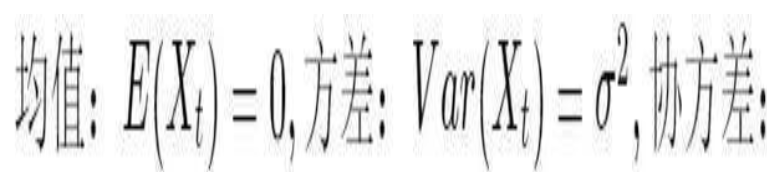白噪声序列的均值和方差为常数，间隔大于0的自协方差都恒等于0，因此是平稳的时间序列。如果白噪声过程中各变量独立并且都服从正态分布，则该序列为高斯白噪声过程（Gussian White Noise）。高斯白噪声序列是强平稳的时间序列，并且各期之间不仅不相关还相互独立。

如果一个时间序列满足高斯白噪音过程，则无法根据过去信息来预测未来。比如非平稳时间序列：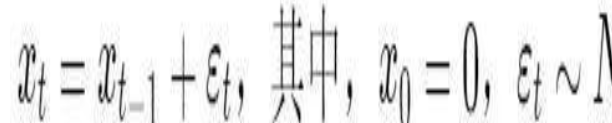这里的随机扰动是服从正态分布的纯随机序列，即为纯随机变量的加总，所以被成为随机游走过程（Random Walk）。根据有效市场假说原理，股票价格是随机游走的，因此是无法被预测的。

白噪声检验（Ljung-Box检验）

如果一个时间序列是纯随机游走的，意味着它的每一次新的变化都无迹可寻，无法从中捕捉对预测有用的信息。换句话说，纯随机时间序列是没有分析和预测的价值的。那么如何检验和判断一个时间序列是否为纯随机序列呢？一般可用Ljung-Box检验方法（简称LB检验）进行统计检验，检验的统计量为Q统计量：

其中，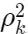是序列的k阶自相关系数，n是整个序列中的观测值个数，m是滞后阶数。当序列存在自相关时，其自相关系数较大，对应的

也较大，相反，当序列为随机序列、无自相关时，序列的自相关系数不会显著地异于0，则

会很小。检验一个时间序列在m阶内是否为白噪声，只有当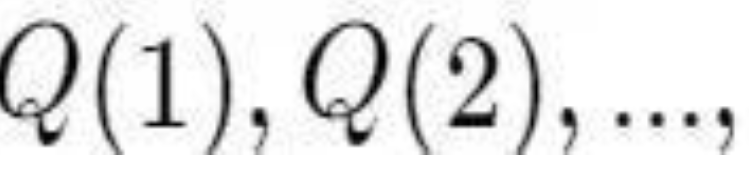m个Q统计量均小于对应的分布的临界值时，才能说明该序列在所检验的m阶内是纯随机的。在实际应用中，LB检验的原假设为所检验序列是纯随机序列，当LB检验统计量对应的p值大于所设定的显著性水平（如5%，1%，0.5%等）时，接受原假设，认为所检验序列为白噪声序列，反之拒绝原假设，认为序列是非白噪声序列。

05 Python模拟白噪音和平稳性检验

#引入statsmodels和scipy.stats用于画QQ和PP图

import scipy.stats as scs

import statsmodels.api as sm

def ts_plot(data, lags=None,title=''):

if not isinstance(data, pd.Series):

data = pd.Series(data)

with plt.style.context('bmh'):

fig = plt.figure(figsize=(10, 8))

layout = (3, 2)

ts_ax = plt.subplot2grid(layout, (0, 0)) acf_ax = plt.subplot2grid(layout, (1, 0))

pacf_ax = plt.subplot2grid(layout, (1, 1))

qq_ax = plt.subplot2grid(layout, (2, 0))

pp_ax = plt.subplot2grid(layout, (2, 1))

data.plot(ax=ts_ax)

ts_ax.set_title(title+'时序图')

smt.graphics.plot_acf(data, lags=lags, ax=acf_ax, alpha=0.5)

acf_ax.set_title('自相关系数')

smt.graphics.plot_pacf(data, lags=lags, ax=pacf_ax, alpha=0.5)

pacf_ax.set_title('偏自相关系数')

sm.qqplot(data, line='s', ax=qq_ax)

qq_ax.set_title('QQ 图')

scs.probplot(data, sparams=(data.mean(), data.std()), plot=pp_ax)

pp_ax.set_title('PP 图')

plt.tight_layout()

return

#Q-Q图的结果与P-P图非常相似，只是P-P图是用分布的累计比，而Q-Q图用的是分布的分位数来做检验

#和P-P图一样，如果数据为正态分布，则在Q-Q正态分布图中，数据点应基本在图中对角线上

模拟白噪声过程

#使用numpy简单模拟白噪声过程

np.random.seed(1)

# plot of discrete white noise

randser = np.random.normal(size=500)

ts_plot(randser, lags=30,title='白噪声')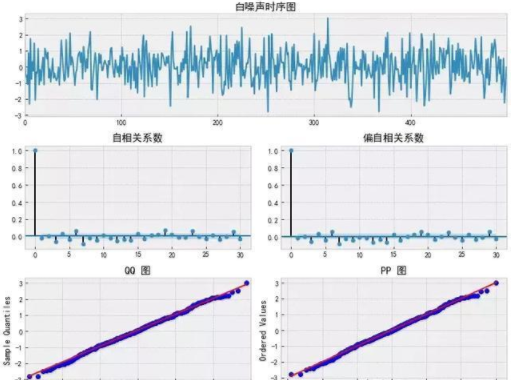可以看到过程是随机且在0附近波动。ACF和PACF显示没有明显的序列相关。要记住，由于是正态分布采样的结果，我们应该在自相关图中看到大约5％的显著性。最下面，QQ图和概率图是比较数据的概率分布和其他理论的分布。在这里，理论分布是标准正态分布，因此我们的数据是正态分布，符合高斯白噪声。随机游走：随机游走是时间序列 的模型：=−1+， 是离散的白噪声序列。随机游走是不平稳的，因为协方差是和时间相关的。如果我们建模的时间序列是随机游走的，那么它是不可预测的。

模拟随机游走过程

#从标准正态分布采样模拟一个随机游走

np.random.seed(2)

n_samples = 1000

x = w = np.random.normal(size=n_samples)

for t in range(1,n_samples):

x[t] = x[t-1] + w[t]

ts_plot(x, lags=30,title='随机游走')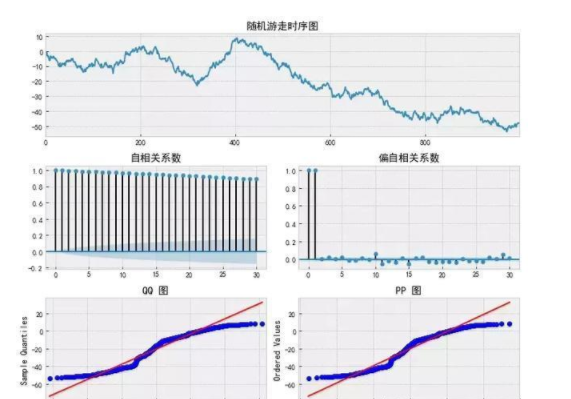明显看出序列是不平稳的。随机游走模型是 =−1+，移项可以得到−−1= 。因此，随机游走的一阶差分应该等于白噪声，可以对时间序列使用 np.diff() 函数看它是否成立。

# First difference of simulated Random Walk series

ts_plot(np.diff(x), lags=30)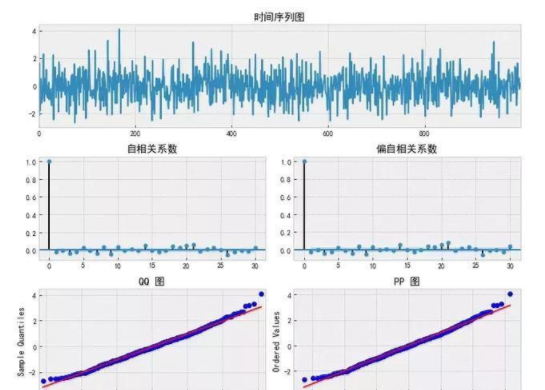应用实例：对沪深300近三年来数据进行平稳性检验分析

#沪深300近三年价格数据

data=pd.DataFrame(df,columns=['close'])

#对数收益率

data['logret']=np.log(data.close/data.close.shift(1))

#普通收益率

data['ret']=data.close/data.close.shift(1)-1

data=data.dropna()

图形观察判断平稳性

#沪深300股价的平稳性

#观察时序图

ts_plot(data.close,lags=30,title='沪深300股价')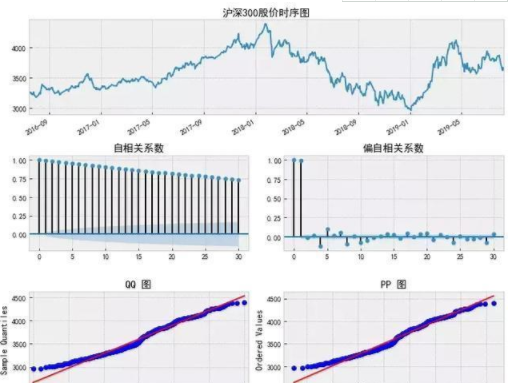上图显示，沪深300近三年股价走势存在明显递增、递减或周期性波动，该时间序列很可能是不平稳的；自相关系数呈现缓慢减小过程， 而偏自相关系数一阶等于1，然后迅速减小，由此可以初步判断其价格不符合平稳性时间序列特征。此外，从QQ图和PP图（二者类似）上不难看出，沪深300价格时间序列不符合正态分布。

#沪深300收益率,对数收益率与算术收益率差异不是很大

ts_plot(data.logret,lags=30,title='沪深300收益率')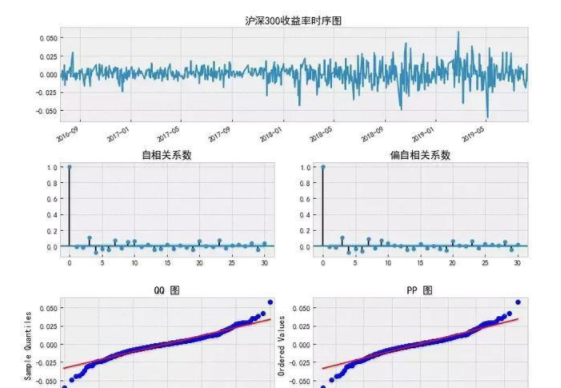沪深300对数收益率时序图围绕某一水平线上下以大致相同的幅度波动，比较像白噪声过程，而自相关与偏自相关系数快速减小至0附近或在某一阶后变为0，从图形上观察应该是平稳过程。从QQ图和概率图上看，该过程很像标准正态分布但是存在厚尾，并且在ACF和PACF图上有一些重要的序列相关性，这意味着应该有更好的模型去描述真实的价格变化过程，为后面时间序列建模设下铺垫。

单位根检验

#这些方法得到的结果是一致的

(-2.050712363785268,

0.26478930749812246,

9,

739,

{'1%': -3.439229783394421,

'5%': -2.86545894814762,

'10%': -2.5688568756191392},

7444.231922186031)

输出结果依次为检验的统计量值、p-value、滞后阶数、自由度等信息,其中检验统计量为-2.05，远大于10%的临界值-2.56，实际上p值为0.2648，远大于0.1，因此我们无法拒绝原假设（原假设是存在单位根），认为该时间序列是非平稳的。

#模块一样，只是引用方式使用了api

import statsmodels.api as sm

(-2.050712363785268,

0.26478930749812246,

9,

739,

{'1%': -3.439229783394421,

'5%': -2.86545894814762,

'10%': -2.5688568756191392},

7444.231922186031)

import statsmodels.tsa.api as smt

(-2.050712363785268,

0.26478930749812246,

9,

739,

{'1%': -3.439229783394421,

'5%': -2.86545894814762,

'10%': -2.5688568756191392},

7444.231922186031)

(-2.050712363785268,

0.26478930749812246,

9,

739,

{'1%': -3.439229783394421,

'5%': -2.86545894814762,

'10%': -2.5688568756191392},

7444.231922186031)

#arch包在后续建模中将会运用到

Augmented Dickey-Fuller ResultsTest Statistic-2.051P-value0.265Lags9

Trend: Constant

Critical Values: -3.44 (1%), -2.87 (5%), -2.57 (10%)

Null Hypothesis: The process contains a unit root.

Alternative Hypothesis: The process is weakly stationary.

结果与上述导入方法是一致的，不过arch包的输出结果比较直观。

#下面沿用arch包的单位根检验函数对沪深300收益率进行单位根检验

Augmented Dickey-Fuller ResultsTest Statistic-8.586P-value0.000Lags8

Trend: Constant

Critical Values: -3.44 (1%), -2.87 (5%), -2.57 (10%)

Null Hypothesis: The process contains a unit root.

Alternative Hypothesis: The process is weakly stationary.

展开全文Python
• 时间序列学习（1）：平稳性、自相关性1、基本统计概念2、啥是时间序列3、平稳性4、自相关性5、相关图 做量化始终逃不掉对时间序列的分析。这个系列笔记是作为小白的我对时间序列分析基础知识的整理。不到之处，多多...python 机器学习
• 相关性检验和独立性检验

千次阅读 2019-04-13 23:29:00
独立性检验表明的是两者是否有关系，相关性检验说明两者成什么样的关系，无论是否有关系都可以表示出回归方程 1 相关性检验 简单相关系数：度量定量变量间的线性相关关系(非相关性) 复相关系数：因变量与多个变量...
• 1.相关性分析        相关性分析是指对两个或多个具备相关性的变量元素进行分析，从而衡量两个变量因素的相关密切程度。相关性的元素之间需要存在一定的联系或者概率才可以进行...
• R语言相关或者部分相关性系数计算实战：使用psych包计算（Partial Correlation）相关或者部分相关性系数并用建设检验检验相关性的显著性 目录 R语言相关或者部分相关性系数计算实战：使用psych包计算...
• 相关性的显著性检验

千次阅读 2017-11-06 12:06:00
x、y：要检验相关性的变量 alternative：指定双侧检验或单侧检验，取值为 two.side,less,或者greater  当研究的假设为总体的相关系数小于 0 时，请使用alternative = “less”  当研究的假设为总体的相关...
• 相关和偏自相关图在时间序列分析和预测中经常使用。这些图生动的总结了一个时间序列的观察值与他之前的时间步的观察值之间的关系强度。初学者要理解时间序列预测中相关和偏自相关之间的差别很困难。在本教程中，...python 自相关
• 相关性表示的是两个观测的数据向量之间的变化关系。一般来讲研究对象(样品或处理组)之间使用距离分析，而元素(物种或环境因子)之间进行相关性分析。两个变量之间的相关性可以用简单相关系数(例如皮尔森相关系数等)...多元相关性分析
• 列明显错误的是( )A 、ρ＝0.8，DW ＝0.4B 、ρ...原始数据三、多项选择题1、DW 检验不适用一下列情况的序列相关检验()A 、高阶线性回归形式的序列相关B 、一阶非线性回归的序列相关C 、移动平均形式的序列相关D...
•  最近因为工作的关系需要使用matlab作为数据统计的工具，其中一个关键是使用其相关函数获得数据的估计。自己只在本科时候马马虎虎地学习了一点matlab，这次仗着有C/C++的基础迅速地过了一遍自己需要的matlab的...matlab 自相关检测
• 如图，我用acf()函数对一时间序列，做相关检验，R出来的相关系数和相关图要怎么分析？求解！！ 看图中的横轴lag表示滞后阶数，纵轴表示对应各阶的相关系数，0阶滞后表示对...
• 误差相关性与R语言

千次阅读 2020-03-24 09:09:52
文章目录误差自相关性检验D-W检验拉格朗日乘数检验举个例子1(DW检验)举个例子2(拉格朗日乘数检验)误差相关性处理方法Durbin两步估计法举个例子(Durbin两步估计法) 误差自相关性检验 D-W检验 DW取值在不同区间内的...DW检验
• (3)检验自相关性 ①残差图分析：在方程窗口中点击Resids按钮，所显示的残差图(图5.3.7所示)表明e呈现有规律的波动，预示着可能存在自相关性。 图5.3.7 残差图 运用GENR生成序列E，观察E，E(-1)图形(见图5.3.8)。 图...
• z分数  z分数（z-score），也叫标准分数（standard score）是一个数与平均数的差再除以标准差的过程。 正太Q-Q图 茎叶图 茎叶图读法如下图； 第一行：B区域为46，48；... 单样本T检验、独立样本...
• R语言相关性分析

万次阅读 多人点赞 2019-11-12 22:52:00
文章目录@[toc]Pearson相关系数(积差相关系数)适用条件Spearman等级相关系数适用条件Kendall's Tau相关系数适用条件相关适用条件R语言实现Pearson、Spearman、Kendall示例相关相关性显著性检验相关性可视化在...R语言 相关性分析 协方差
• 小二乘法检验matlab代码地面真相 用于分析语言特征与 GroundTruth 研究的心理健康分数之间的相关性的代码 概述：为了调查语言特征与抑郁症状、广泛性焦虑和自杀意念之间的关联，我们从个人博客内容中提取了语言...
• 另一个非常重要的函数是部分相关函数，其中消除了先前的滞后对当前区间的影响，只保留了当前区间滞后的影响来分析，例如：第四个滞后的偏自相关将消除第一、第二和第三个滞后的影响。部分相关图如下： 可以看到...
• 目录：前言相关或复相关意义与用途分析方法：1、 样本相关系数矩阵、相关系数检验2、 复相关分析3、 决定系数 （RMSE的介绍）小结一、前言：继上一篇文章，继续探讨相关性分析，这次不再是两个变量，而是3个或者...
• r语言实现相关分析和相关分析 相关分析 为什么要做相关分析： 对数据进行建模前首先要对数据有一个大致的理解，相关分析可以帮助人们看出数据是否平稳，时间序列是否存在某种变化的趋势。 相关简介： ...数据分析 r语言 数据建模
• [偏相关分析]偏相关系数计算及假设检验

万次阅读 多人点赞 2017-10-30 10:54:41
1、相关分析通过计算两个变量之间的相关系数，分析变量间线性相关的程度，在多元相关分析中，由于受到其他变量的...2、当其他变量被固定住，即将他们控制起来后，给定的任意两个变量之间的相关系数叫相关系数，相关偏相关系数 假设检验
• R之相关性的显著性检验

万次阅读 多人点赞 2017-12-26 19:26:01
# 其中的x和y为要检验相关性的变量，alternative则用来指定进行双侧检验或单侧检验（取值 # 为"two.side"、"less"或"greater"），而method用以指定要计算的相关类型（"pearson"、 # "kendall"或"spearman"）。 #当...
• (由Python大本营付费下载视觉中国)作者 |Leandro Rabelo译者|李洁整理|Lemonbit出品|Python数据之道本文内容较长，较为详细的阐述了进行时间序列预测的步骤，有些内容可能暂时用不到或者看不懂，但不要紧，知道有...
• R语言进行的变量相关性显著性检验 在计算好相关系数以后，如何对它们进行统计显著性检验呢？ 常用的原假设为变量间不相关（即总体的相关系数为0）。可以使用cor.test()函数对单个的Pearson、Spearman和Kendall相关...
• 两个变量与因变量相关性分析提问：用SPSS一个分析,有一个因变量和N个变量,先做相关性发现有很多变量与因变量有关,相关性也比较高.继续说,但是再做多重回归方程的时候只有3个因变量入选,其他都被排除了,那在写......